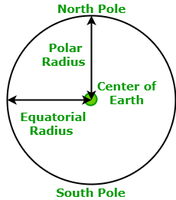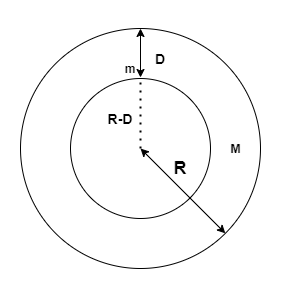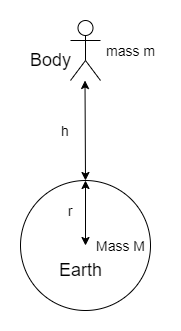Acceleration due to Gravity

• Last Updated : 14 May, 2021

During skydiving when a skydiver jumps from the plane there is a sudden increase in the fallen speed, the increase is because of the acceleration due to gravity, and the force that attracts the body i.e. the gravitational force. Also, when the skydiver opens the parachute, the parachute opposes the gravity and decreases the speed, so that the person landed safely. It is also observed that when a ball is moving in upwards direction exhibit less speed than when it comes down. This is all because of the acceleration, which is produced due to the force of gravity.

Gravitational Force

The attraction of objects towards the earth is known as the force of gravity or gravity. Every object in the universe whether how big or small exerts a force on every other object, Thus this force is known as gravitational force.

e.g.: The force acting between any two books or any two objects on the Earth’s surface.

Moreover, the gravitational force also defined as any force that attracts two objects with a mass. Furthermore, this gravitational force attracts because it always tries to pull masses together, and it never pushes them apart as it is an attractive force. Additionally, every object including you pulls attracted by every other object in the entire universe and this is called Newton’s Universal Law of Gravitation or the gravitational formula.

It is stated as:where, FG is the Gravitational constant,

G is the Gravitational Constant \left(6.67\times10^{-11}\text{N}\cdot\text{m}^2/\text{kg}^2\right),

d is the distance between the two masses,

M and m are the masses of the two objects in contact.

The SI unit of the Gravitational force is Newton (N).

Acceleration due to gravity

When a body is fallen towards the earth it experiences a change in its acceleration due to the earth’s gravitational force. This acceleration is called acceleration due to gravity. This is the acceleration that is attained by an object due to the gravitational force.

The SI unit of acceleration due to gravity (g) is m/s²

Since, the acceleration due to gravity has a magnitude as well as direction. Thus, it is a vector quantity.

Its standard value on the surface of the earth at sea level is 9.8 m/s².

Mathematically, the acceleration due to gravity is directly proportional to the mass of the object and inversely proportional to the distance from the centre of mass, so given as:orwhere, G is the gravitational constant,

m is the mass of the body and

r is the distance from the centre.

Derivation for the formula of acceleration due to gravity

According to the second law of motion:

F = ma

But, in case of a free-falling body, the force is equal to the product of the mass of the body and acceleration due to gravity.

F = mg                                                                                                                   ……(1)

But, according to the universal law of gravitation:……(2)

Now, from the equation (1) and (2),Consider for an ideal case the object is placed near to earth therefore the distance between earth and object will be radius of earth, so replace d with r and rearranging the above expression for g as:Calculation of the value of acceleration due to gravity

The acceleration due to gravity is stated as:Here, substitute 6.67 × 10-11 Nm2 kg-2 for G, 6 × 1024 kg for M and 6.4 × 106 m for r in the above expression to calculate g at the surface of Earth.Factor affecting acceleration due to gravity

• The Shape of Earth: As it is known that the shape of the earth is not spherical it’s quite oval so the gravitational force is different at different places. The force of attraction is maximum at the pole of the earth approximately 9.82 m/s2 as the radius of the earth is minimum at the pole. While the force of gravitation is minimum at the equator of the earth approximately 9.78 m/s2 as the radius of the earth is maximum at the equator• Altitude: When a body moves away from the surface of the earth the force of attraction decreases as the distance between the earth and the body increases.
• Depth: When a body is put inside the earth’s surface the acceleration due to gravity becomes less.

Effects on g due to depth DConsider an object of mass m inside the earth of mass M at depth D.

The acceleration due to gravity at the surface of Earth in terms of density is:

g = 4/3 x  πρ x RG

At depth D

gD = 4/3 x  πρ x (R-D)G

on dividing both equations we get

gd = g x πρ x (R-D)

Now two cases can be possible:

Case 1: If depth D is equal to the radius of the earth i.e. D = R, then:

gd = 0

Case 2: If depth D = 0, i.e. the object is at the surface of earth, then

gd = g

Effects on g due to height hIf an object is placed at a certain height h then the value of d will become (r+h) so,

gh = GM/(r+h)2

= GM/r2 (1+h/r)2

= (GM/r2)/(1+h/r)2

= g / (1+h/r)2                                       (Since, g = GM/r2)

When h is much lesser than radius of earth, the value of g at height h is given by:

gh = g/(1 – 2h/r)

Sample Problems

Here are some problems solved which are based on the topics discussed above.

Solution:

Given that,

The height of the body, h is 2 x 106 m.

The mass of the body, m is 20 kg.

By the formula for g at a height h is:

gh = g / (1+h/r)2

Here, g is the acceleration due to gravity, h is the height and r is the radius of the Earth.

Substitute 2 x 106 m for h, 9.8 ms-2 for g and 6.4 x 106 m for r in the above expression to calculate gh.

gh = 9.8 /(1+(2×106 / 6×106))

= 7.35 ms-2

Hence, the acceleration due to the gravity of the body at height h is 7.35 ms-2.

Solution:

The acceleration due to gravity is:

g=GM/r2

Here, G is the Gradational constant. M is the mass and r is the radius of the Earth.

The acceleration due to gravity on Earth is:

ge = G x Me/re2                                                                                                         ……(1)

The acceleration due to gravity on Moon is:

gm = G x Mm/rm2                                                                                                      ……(2)

Divide equation (1) by equation (2) as:

ge/gm = (Me x rm2)/(Mm x re2)

Substitute the given values in the above expression, to calculate the ratio of acceleration due to gravity on earth w.r.t. to the moon.

ge/gm = 6.062 ≈ 6

Hence, the ratio of acceleration due to gravity on earth w.r.t. to the moon is equal to 6:1.

Solution:

The acceleration due to gravity in terms of density is:

g=4/3 x πρ x RG

Here, ρ is the density, R is the radius and G is the gravitational constant.

And, at depth d is:

gd = 4/3 x πρ x (R-d)G

= 4/3 x 5515 x (6 x 106 – 1.5 x 106) x 6.67 x 10-11

= 0.206 m/s2

Hence, the acceleration due to the gravity of the body is equal to 0.206 m/s2.

Solution:

The acceleration due to gravity at a height h is:

gh = g / (1+h/R)2

Now, it is given that: h = R/2.

Therefore, the above expression becomes:

gh = g/(1+R/2R)2

= g/ (3/2)2

= 4/9 x g

Hence, the values of g decreases by 4/9 times.

Solution:

Given that,

The radius of the planet, rp = 2r.

The mass of the planet, Mp = 2M

The formula to calculate the acceleration due to gravity of the planet,

gp = GMp / rp2

= (2GM) / (2r)2

= (1/2)  GM/r

= 1/2 x g                        (Since, g=GM/r2 )

Hence, the acceleration due to gravity on the planet is half times the acceleration due to gravity on earth.

My Personal Notes arrow_drop_up